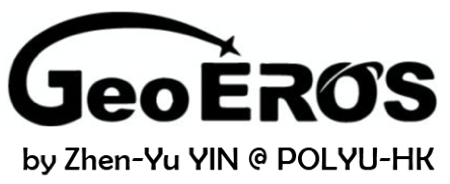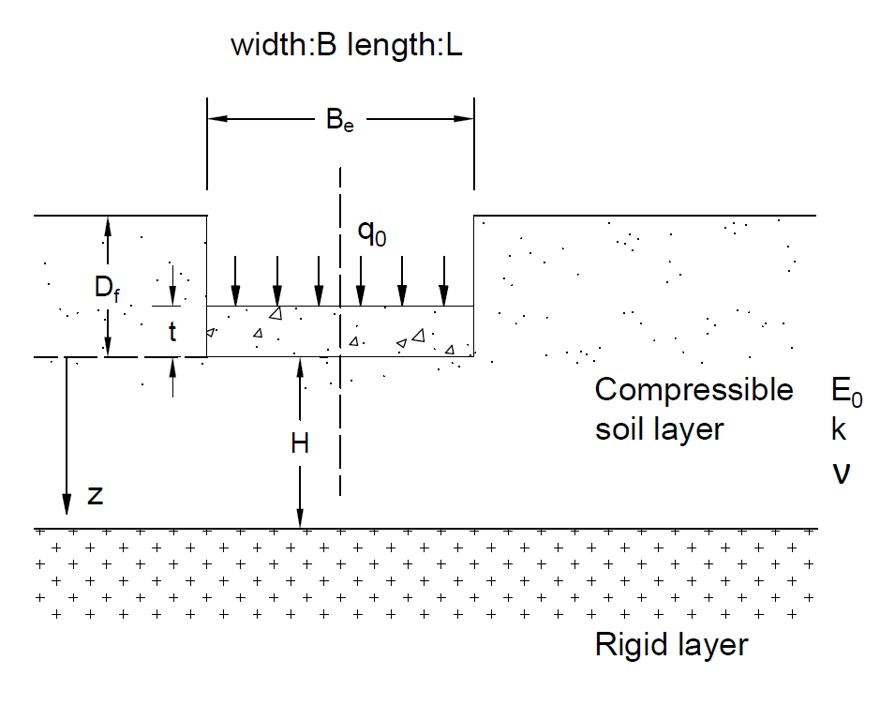# Shallow Foundation Tool (Se)## Geometry of Shallow Foundation (elastic settlement)## Tips

• This tool is for calculating elastic settlement of the soil below the shallow foundation, as shwon in the figure above.
• The Bowles method (only for the center point) and the Mayen&Poulos method (for the center and corner point) are used to calculate the elastic settlement.
• Averaged Young's modulus Eav is only used by Bowles method.
• Thickness of the foundation t, Young's modulus of foundation material Ef, Young's modulus of soil at the depth of foundation bottom E0,and rate of change of the soil Young's modulus with depth k are only used by Mayen&Poulos method.
• The unit of input Ef is GPa, and the unit of input E0 and Eav is kPa.
• By Bowles method, the elastic settlement of center Se1 is for perfectly flexible foundation. The elastic settlement of rigid foundation Se(rigid) can be obtained by Se(rigid) ≈ 0.93Se1.
• All the input parameters should be positive.
• For "nan", "0" or "inf" displayed in Results, please check your input parameters.

B (m)
L (m)
Df (m)
H (m)
q0 (kN/m2)
ν
Eav (kPa)
t (m)
Ef (GPa)
E0 (kPa)
k (kPa/m)

## Output Results

• Bowles Method
Depth factor
If =
Shape factor of center
Is1 =
Shape factor of corner
Is2 =
Elastic settlement of center
Se1 (m) =
Elastic settlement of corner
Se2 (m) =
• Mayne&Poulos Method
Equivalent width
Be (m) =
Influence facotr of E with depth
IG =
Correction factor of foundation rigidity
IF =
Correction factor of embedment
IE =
Elastic settlement of center
Se (m) =